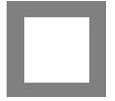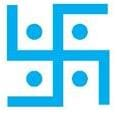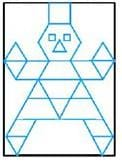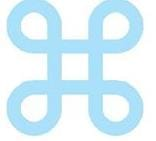# Important Questions Test: Symmetry

## 20 Questions MCQ Test NCERT Mathematics for CAT Preparation | Important Questions Test: Symmetry

Description
Attempt Important Questions Test: Symmetry | 20 questions in 20 minutes | Mock test for Class 6 preparation | Free important questions MCQ to study NCERT Mathematics for CAT Preparation for Class 6 Exam | Download free PDF with solutions
QUESTION: 1

Solution:
QUESTION: 2

Solution:
QUESTION: 3

### What is the other name for a line of symmetry of a circle?

Solution:

A diameter divides the circle into 2 equal parts. So, it can be considered as a line of symmetry.

QUESTION: 4

Which of these letters of the English alphabet has reflectional symmetry about a vertical mirror?

Solution:

U appears the same in mirror too.

QUESTION: 5

Find the number of lines of symmetry in regular hexagon.

Solution:
QUESTION: 6

Letter ‘A’ of the English alphabet have reflectional symmetry (i.e., symmetry related to mirror reflection) about.

Solution:
QUESTION: 7

Letter ‘C’ of the English alphabet have reflectional symmetry (i.e., symmetry related to mirror reflection) about.

Solution:
QUESTION: 8

Letter ‘E’ of the English alphabet have reflectional symmetry (i.e., symmetry related to mirror reflection) about.

Solution:
QUESTION: 9

Letter ‘H’ of the English alphabet have reflectional symmetry (i.e., symmetry related to mirror reflection) about.

Solution:
QUESTION: 10

Letter ‘M’ of the English alphabet have reflectional symmetry (i.e., symmetry related to mirror reflection) about.

Solution:
QUESTION: 11

Letter ‘T’ of the English alphabet have reflectional symmetry (i.e., symmetry related to mirror reflection) about.

Solution:
QUESTION: 12

Letter ‘G’ of the English alphabet have reflectional symmetry (i.e., symmetry related to mirror reflection) about.

Solution:
QUESTION: 13

Letter ‘D’ of the English alphabet have reflectional symmetry (i.e., symmetry related to mirror reflection) about.

Solution:
QUESTION: 14

Letter ‘B’ of the English alphabet have reflectional symmetry (i.e., symmetry related to mirror reflection) about.

Solution:
QUESTION: 15

Letter ‘I’ of the English alphabet have reflectional symmetry (i.e., symmetry related to mirror reflection) about.

Solution:
QUESTION: 16

Find the number of lines of symmetry in the below figure:Solution:
QUESTION: 17

Find the number of lines of symmetry in the below figure:Solution:
QUESTION: 18

Find the number of lines of symmetry in the below figure:Solution:
QUESTION: 19

Find the number of lines of symmetry in the below figure:Solution:
QUESTION: 20

Find the number of lines of symmetry in a circle.

Solution:Use Code STAYHOME200 and get INR 200 additional OFF Use Coupon Code